mraa  2.0.0 Low Level Skeleton Library for Communication on GNU/Linux platforms
Aio Class Reference

## Detailed Description

This file defines the aio interface for libmraa

/* initialize AIO */
mraa::Aio aio(AIO_PORT);
while (flag) {
std::cout << "ADC A0 read %X - %d" << value << value << std::endl;
std::cout << "ADC A0 read float - %.5f" << float_value << std::endl;
}

## Public Member Functions

Aio (int pin)

Aio (void *aio_context)

~Aio ()

unsigned int read ()

Result setBit (int bits)

int getBit ()

## Constructor & Destructor Documentation

 Aio ( int pin )
inline

Aio Constructor, takes a pin number which will map directly to the board number

Parameters
 pin channel number to read ADC inputs

Here is the call graph for this function: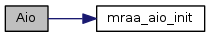Aio ( void * aio_context )
inline

Aio Constructor, takes a pointer to the AIO context and initialises the AIO class. Aio pins are always 0 indexed reguardless of their position. Check your board mapping for details. An arduino style layout will have A0 as pin14 but AIO0.

Parameters
 aio_context void * to an AIO context
 ~Aio ( )
inline

Aio destructor

Here is the call graph for this function:## Member Function Documentation

 unsigned int read ( )
inline

Read a value from the AIO pin. By default mraa will shift the raw value up or down to a 10 bit value.

Exceptions
 std::invalid_argument in case of error
Returns
The current input voltage. By default, a 10bit value

Here is the call graph for this function: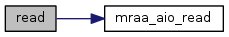float readFloat ( )
inline

Read a value from the AIO pin and return it as a normalized float.

Exceptions
 std::invalid_argument in case of error
Returns
The current input voltage as a normalized float (0.0f-1.0f)

Here is the call graph for this function: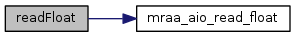Result setBit ( int bits )
inline

Set the bit value which mraa will shift the raw reading from the ADC to. I.e. 10bits

Parameters
 bits the bits the return from read should be i.e 10
Returns
mraa::Result type

Here is the call graph for this function: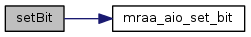int getBit ( )
inline

Gets the bit value mraa is shifting the analog read to.

Returns
bit value mraa is set return from the read function

Here is the call graph for this function: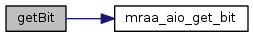The documentation for this class was generated from the following file: